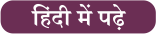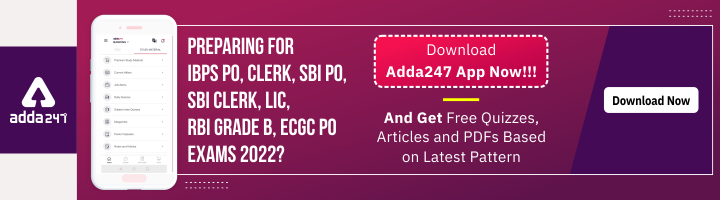Latest Banking jobs   »   Alphabet Series Reaoning.

# Alphabet Series Reasoning: Questions, Concept, Solved Example

Alphabet Series Reasoning:  Alphabet Series is one of the most scoring topics in the reasoning section. Generally, 3-4 questions are asked from the alphabet series in the banking exams which a candidate can do easily but what matters is how quickly the question is done by you as every minute is important. The Alphabet series questions are also asked in other competitive exams as well like SSC, Insurance, Railway and  state exams. To solve these kinds of questions, candidates are required to use their analytical power. In this article, we have discussed the Alphabet series reasoning questions, concepts and solved example.

## What is Alphabet Series in Reasoning?

In the reasoning section, one of the most scoring sections is the alphabet series. In the alphabet series section, a string of alphabets, either in a single file or in combination form a sequence. These types of questions come together following a definite rule. The aspirants is expected to detect this rule and answer the questions at the end. Most of the time an alphabet series or sequences will be asked in a set of 5 questions and candidates will have to answer the question asked based on these sets of questions.

## Alphabet Series Reasoning: Tips, Tricks & Concepts

Candidates can check here some tips and tricks of the Alphabet Series in the Reasoning Ability section.

• In the alphabet series Left – Left from the left end, Right – Right from the right it means right from the right end of the series.
• Sometimes series with either missing elements or questions with sub parts may be asked which comprise only English Alphabets and no numbers set in a specific pattern.
• In the question where the number of words is starting with vowels after replacing vowels of each word with its next letter means we have to replace each vowel with its next word and answer the questions.
• Right + Left means from the right end.
• Left+Right means form the from the left end.## Alphabet Series Reasoning: Types, Questions & Answers

Directions (1-5): Following questions are based on the five words given below, Study the following words and answer the following question:

MINTS           RAGSE           CULTS           NIGAS            PEMTO

Q1. If the given words are arranged in the order as they appear in a dictionary from left to right, which of the following word will be the fifth from the left end?

(a) MINTS

(b) RAGSE

(c) CULTS

(d) NIGAS

(e) PEMTO

Q2. If the letters of the given words are arranged in alphabetical order, then within the word how many meaningful words will be formed?

(a) Four

(b) Three

(c) Two

(d) One

(e) None

Q3. How many letters are there between the first Letter of third word from left end and Last Letter of the second word from right end (According to the alphabetical order)?

(a) Eight

(b) Nine

(c) Ten

(d) Fifteen

(e) Sixteen

Q4. How many vowels are there in between the first letter of first word from left and last letter of last word from right (According to the alphabetical order)?

(a) Two

(b) Three

(c) None

(d) Five

(e) None of these

Q5. How many letters are there between the first letter of last word from left and first letter of the second word from left (According to the alphabetical order)?

(a) Four

(b) Fourteen

(c) Twenty

(d) Six

(e) One

Directions (6-10): Study the following alphabetical sequence and answer the questions following it.

A B B C D E F E I B C A F E C B B A C A O B N U V W

Q6. How many B’s are there in the alphabetical series which are immediately preceded by a

vowel and immediately followed by consonant?

(a) One

(b) Two

(c) Three

(d) More than three

(e) None of these

Q7. If all the vowels are dropped from the series, then which alphabet will be eighth from

the right end?

(a)C

(b)B

(c) N

(d)F

(e) None of these

Q8. How many consonants are there in the alphabetical series which are immediately

preceded by a Vowel?

(a) One

(b) Two

(c) Four

(d) None

(e) More than Five

Q9. If the position of the 1st and the 14th alphabets, the 2nd and the 15th alphabets, and so on up to the 13th and the 26th alphabets, are interchanged, then which alphabet will be 7th to

the right of 10th alphabet from the right end?

(a) A

(b) C

(c) N

(d)B

(e) None of these

Q10. How many total vowels are there in the alphabetical series?

(a) Five

(b)Ten

(c)Three

(d) Nine

(e) None of these

Directions (11-15): Study the following alphabetical sequence and answer the questions following it.

A B B C D E F E I B C A F E C B B A C A O B N U V W

Q11. How many B’s are there in the alphabetical series which are immediately preceded by a vowel and immediately followed by consonant?

(a) One

(b) Two

(c) Three

(d) More than three

(e) None of these

Q12. If all the vowels are dropped from the series, then which alphabet will be eighth from the right end?

(a)C

(b)B

(c) N

(d)F

(e) None of these

Q13. How many consonants are there in the alphabetical series which are immediately preceded by a Vowel?

(a) One

(b) Two

(c) Four

(d) None

(e) More than Five

Q14. If the position of the 1st and the 14th alphabets, the 2nd and the 15th alphabets, and so on up to the 13th and the 26th alphabets, are interchanged, then which alphabet will be 7th to the right of 10th alphabet from the right end?

(a) A

(b) C

(c) N

(d)B

(e) None of these

Q15. How many total vowels are there in the alphabetical series?

(a) Five

(b)Ten

(c)Three

(d) Nine

(e) None of these

SOLUTIONS

Solutions (1-5):

S1. Ans. (b)

Sol. Original Series:         MINTS   RAGSE   CULTS   NIGAS   PEMTO

After Rearrangement:         CULTS   MINTS   NIGAS   PEMTO   RAGES

S2. Ans. (e)

Sol. Original Series:            MINTS   RAGSE   CULTS   NIGAS   PEMTO

After Rearrangement     IMNST      AEGRS     CLSTU     AGINS   EMOPT

S3. Ans. (d)

Sol. Between (C and S) only fifteen letters are there.

S4. Ans. (c)

Sol. Between (M and O) there are No vowels.

S5. Ans. (e)

Sol. Between (P and R) there are only one letters.

Solutions (6-10):

S6. Ans (c)

S7. Ans (a)

S8. Ans (e)

S9. Ans (b)

S10. Ans (b)

Solutions (11-15)

S11. Ans (c)

S12. Ans (a)

S13. Ans (e)

S14. Ans (b)

S15. Ans (b)## FAQs: Alphabet Series Reasoning

Q1. In which exams Alphabet Series Reasoning questions are asked?

Ans. Many prestigious government exams such as SSC, Railway, IBPS PO, Clerk, RRB PO, Clerk, SO, SBI PO, Clerk, SO, UPSC, LIC AAO, ADO, Assistant, etc. ask questions from Alphabet Series Reasoning.

Q2. What is Alphabet Series in Reasoning?

Ans. In the reasoning section, one of the most scoring sections is the alphabet series. In the alphabet series section, a string of alphabets, either in a single file or in combination form a sequence.

Q3. What are the topics of the Series in Reasoning?

Ans. The main topics of series in reasoning are alphabet series, alphanumeric series, mix series, etc.

Q4. Which types of questions are asked in the Alphabetic series in the reasoning ability section?

Ans. Candidates can check the types of questions of the Alphabetic series in the reasoning ability section in the above article.

Recent Posts#### Congratulations!Incorrect details? Fill the form again here

•The Hindu Review June 2022: Download Hin...
•02nd July Daily Current Affairs 2022: To...
•Most Expected GA Questions For IDBI Exec...
•World UFO Day 2022, History & Signif...
•What Is PET Training In IBPS Clerk?
•GA Topper Series: Space Exploration Miss...
•8000+ Government Employees Promoted By C...